Question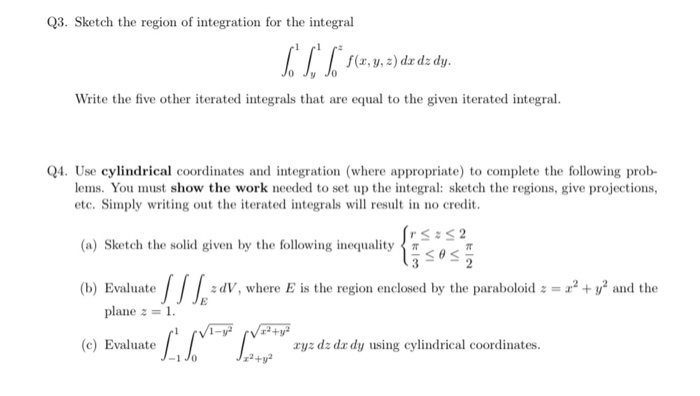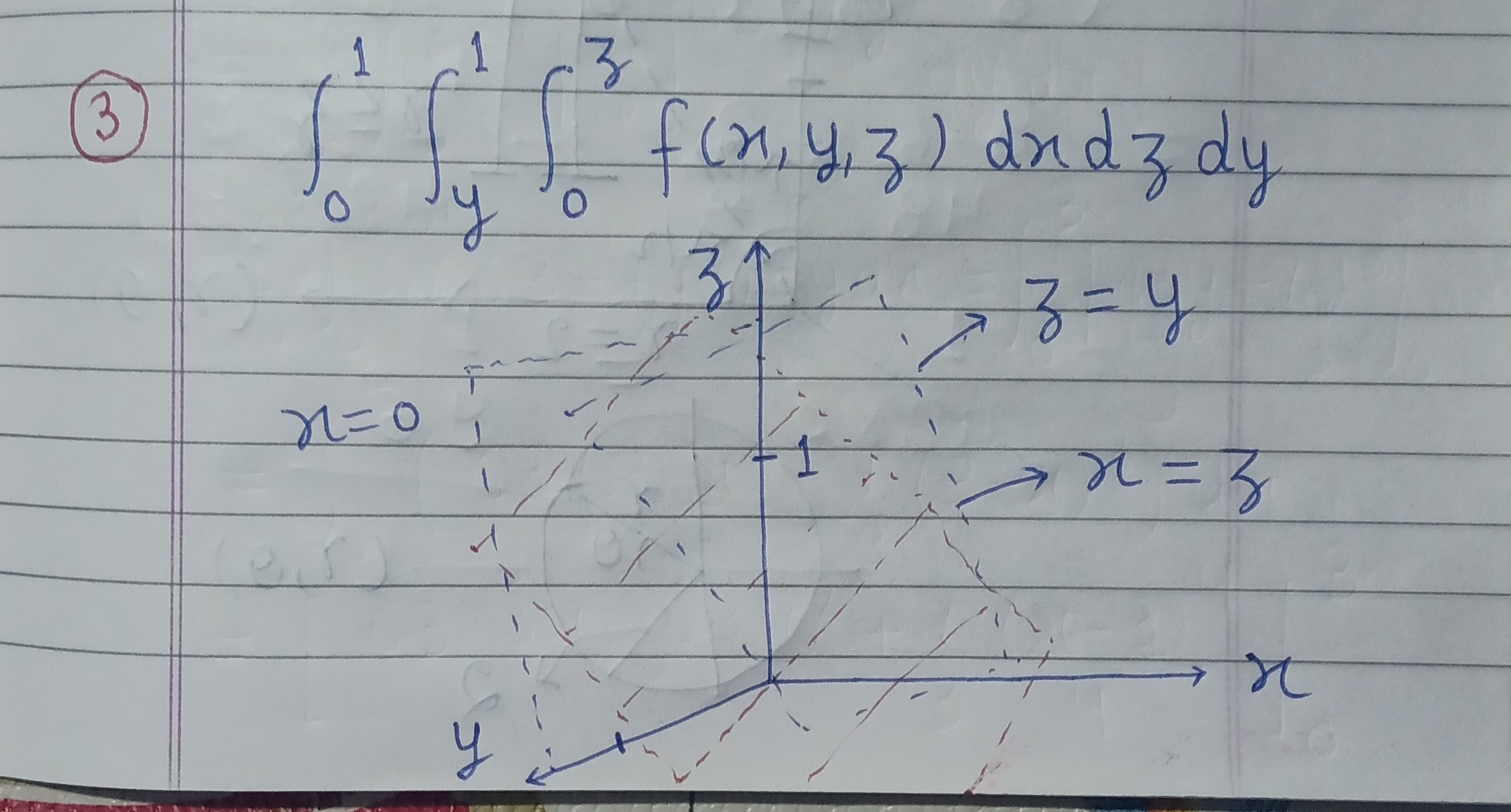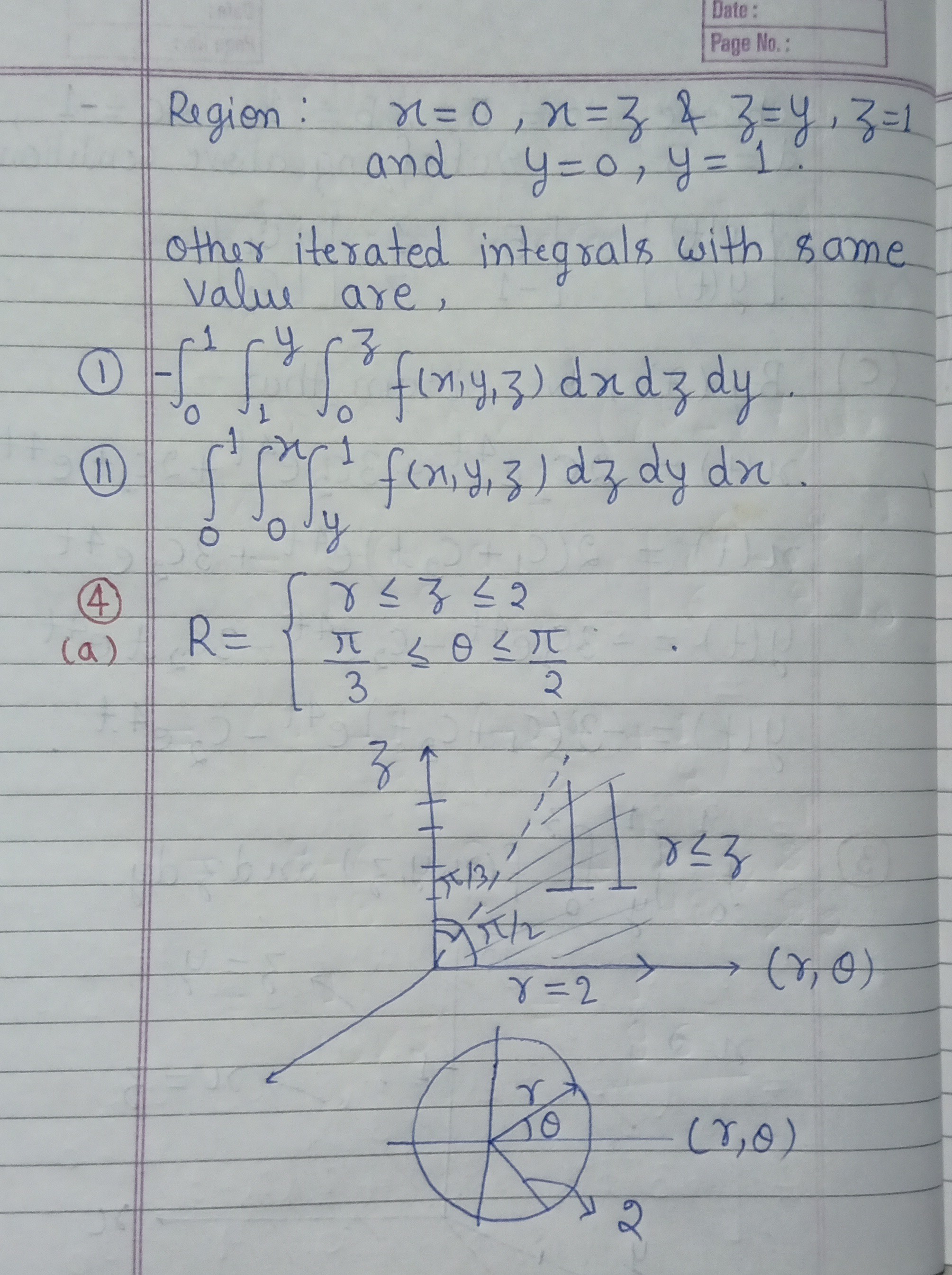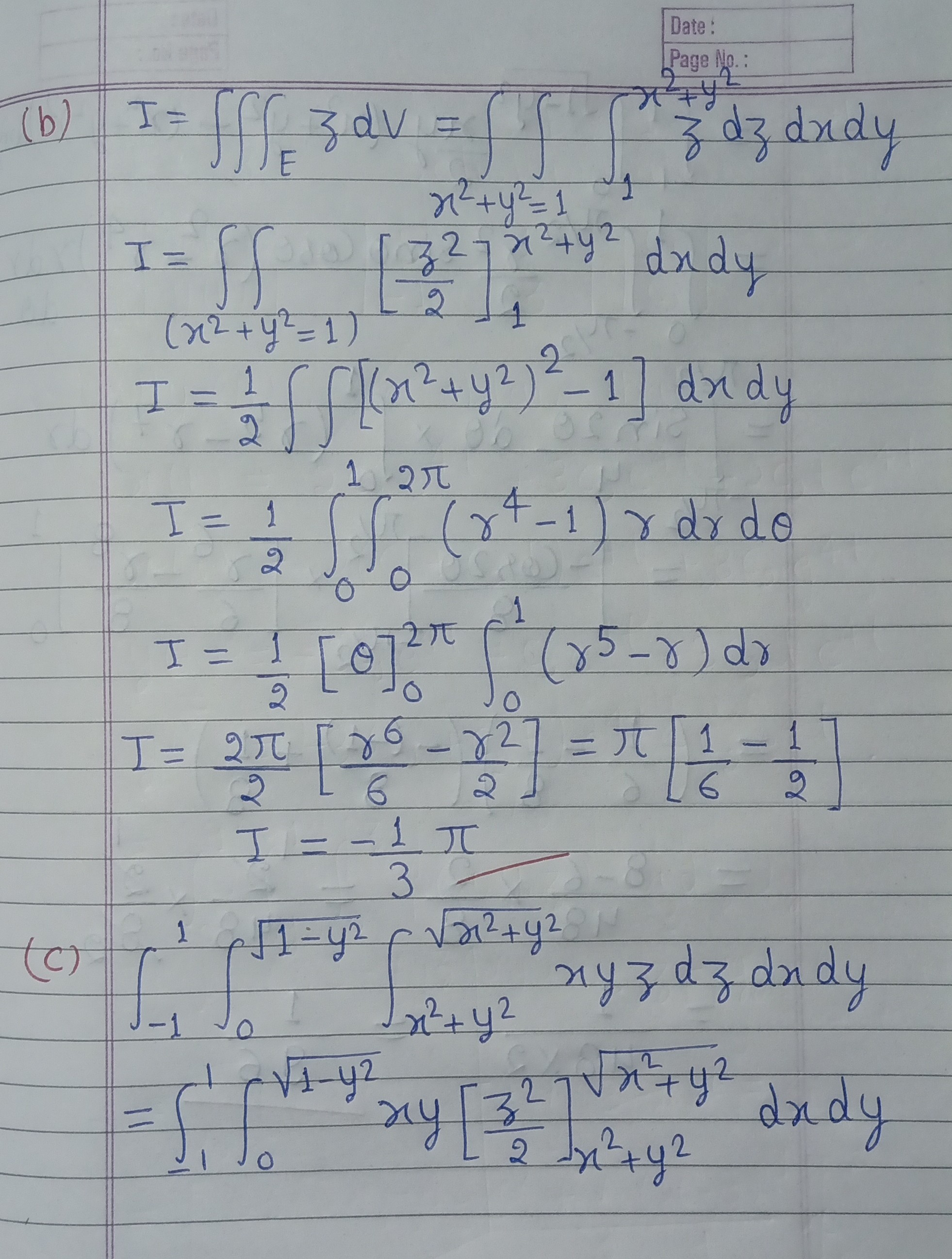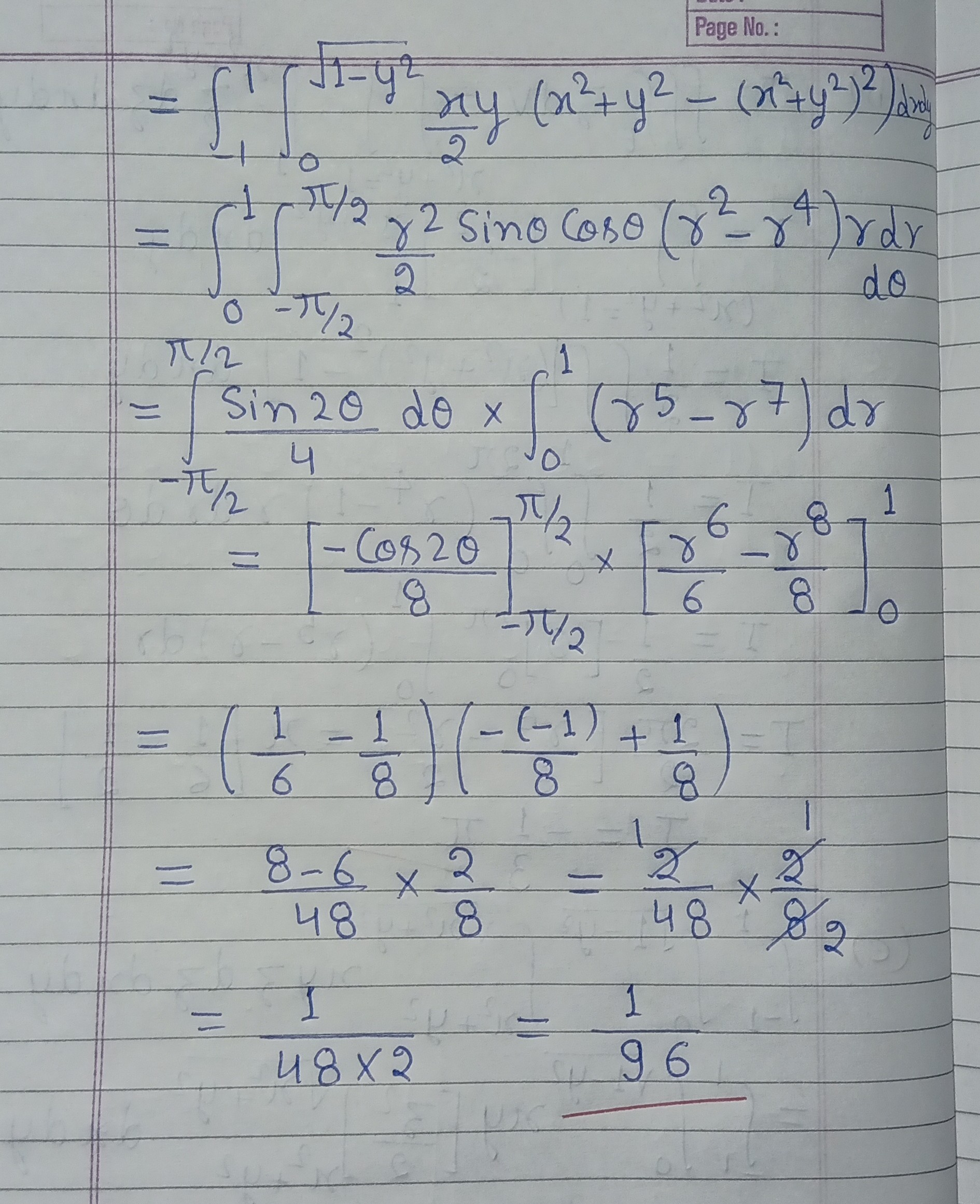#### Earn Coins

Coins can be redeemed for fabulous gifts.

Similar Homework Help Questions
• ### 15. (15 points. (a) Sketch the region of integration for the iterated integral . Lzi?dz dy....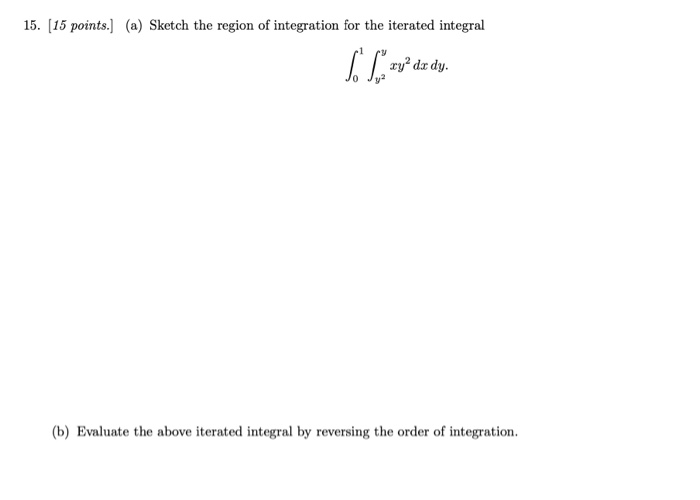15. (15 points. (a) Sketch the region of integration for the iterated integral . Lzi?dz dy. (b) Evaluate the above iterated integral by reversing the order of integration.

• ### The figure shows the region of integration for the integral. fx, y, z dy dz dx 0 Jo Rewrite this ...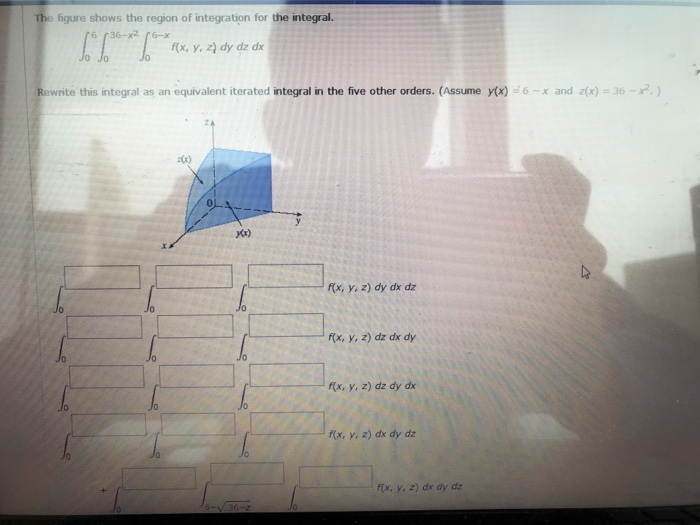The figure shows the region of integration for the integral. fx, y, z dy dz dx 0 Jo Rewrite this integral as an equivalent iterated integral in the five other orders. (Assume yx) 6x and z(x)-36-) x. f(x, y, 2) dy dx dz x, , z) dz dx dy f(x, y, z) dz dy dx f(x, y, z) dx dy dz fx, y, z) dx dy dz J0 Jo Jo f(x, y, z) dz dx dy 0 0 f(x, y,...

• ### 2. Sketch the region of integration for SL to dz dy de and rewrite in the...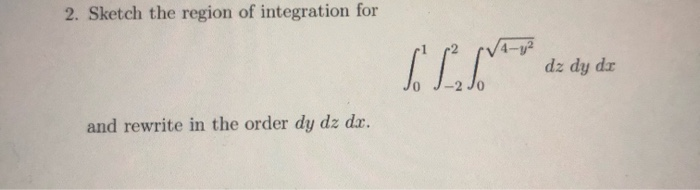2. Sketch the region of integration for SL to dz dy de and rewrite in the order dy dz dx.

• ### 8. Sketch the region of integration and evaluate the integral re dx dy, where G is the region bounded by 0,1, -o,y- 8. Sketch the region of integration and evaluate the integral re dx dy, where...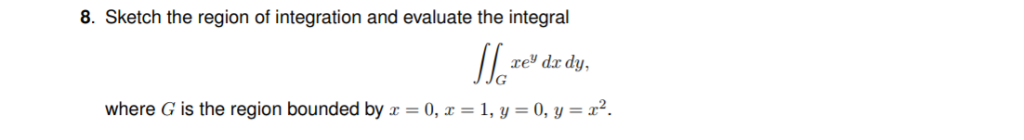8. Sketch the region of integration and evaluate the integral re dx dy, where G is the region bounded by 0,1, -o,y- 8. Sketch the region of integration and evaluate the integral re dx dy, where G is the region bounded by 0,1, -o,y-

• ### please show all work in clean and legible handwriting with all labels and steps that is properly explained for PROBLEMS #1, 2, 3, AND 4. Any incorrect answers and not solving all 4 problems...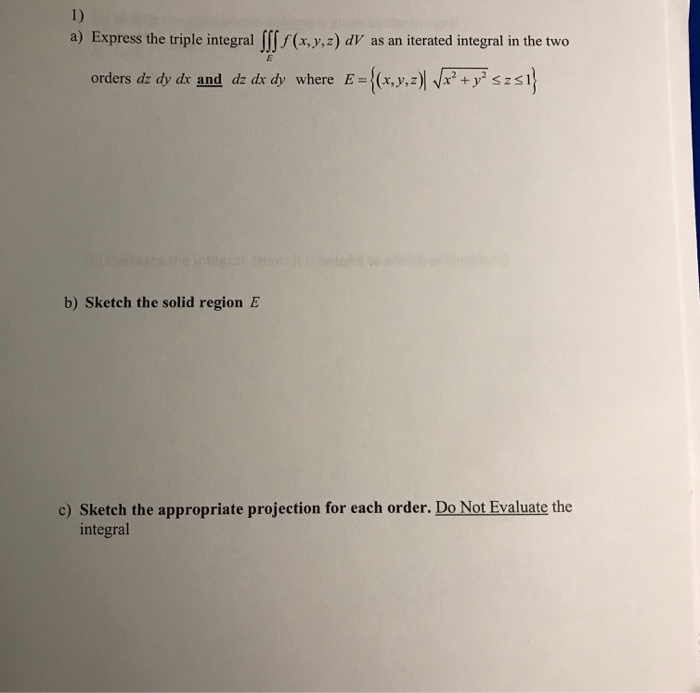please show all work in clean and legible handwriting with all labels and steps that is properly explained for PROBLEMS #1, 2, 3, AND 4. Any incorrect answers and not solving all 4 problems will get an immediate thumbs down because they did not follow directions, thank you 1) Express the triple integral Ⅲf (x,y,z) dV as an iterated integral in the two a) E={(x,y,z)Wr2+yszaj orders dzdy dr and dz dr dy where b) Sketch the solid region E c)...

• ### 1 point) Integratef(x, y, z) 6xz over the region in the first octant (x,y, z 0) above the parabol...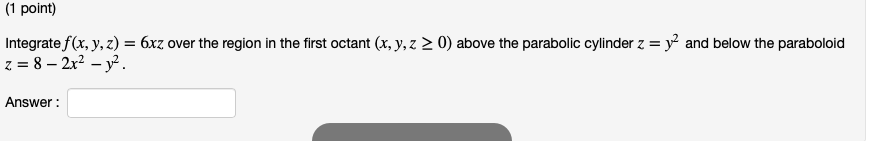Please try helping with all three questions.......please 1 point) Integratef(x, y, z) 6xz over the region in the first octant (x,y, z 0) above the parabolic cylinder z = y2 and below the paraboloid Answer Find the volume of the solid in R3 bounded by y-x2 , x-уг, z-x + y + 24, and Z-0. Consider the triple integral fsPw xyz2 dV, where W is the region bounded by Write the triple integral as an iterated integral in the order...

• ### 6. (4 pts) Consider the double integral∫R(x2+y)dA=∫10∫y−y(x2+y)dxdy+∫√21∫√2−y2−√2−y2(x2+y)dxdy.(a) Sketch the region of integration R in Figure 3.(b)...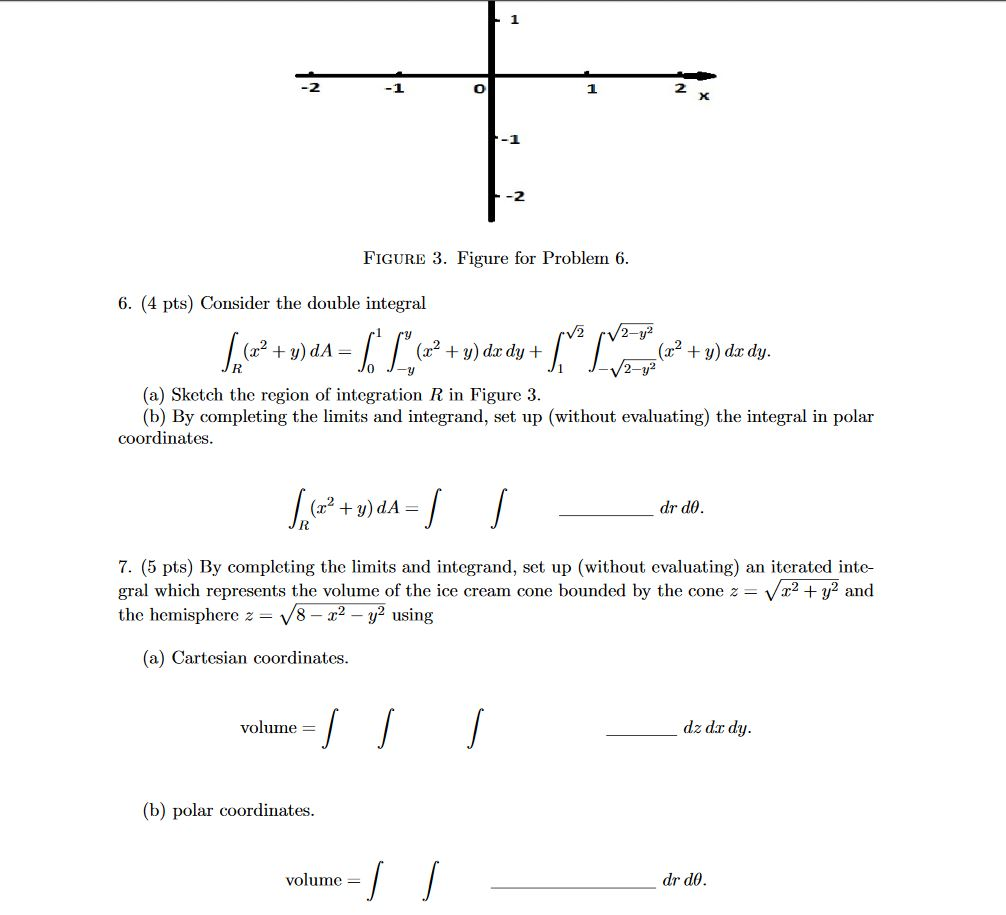6. (4 pts) Consider the double integral∫R(x2+y)dA=∫10∫y−y(x2+y)dxdy+∫√21∫√2−y2−√2−y2(x2+y)dxdy.(a) Sketch the region of integration R in Figure 3.(b) By completing the limits and integrand, set up (without evaluating) the integral in polar coordinates.∫R(x2+y)dA=∫∫drdθ.7. (5 pts) By completing the limits and integrand, set up (without evaluating) an iterated inte-gral which represents the volume of the ice cream cone bounded by the cone z=√x2+y2andthe hemisphere z=√8−x2−y2using(a) Cartesian coordinates.volume =∫∫∫dz dxdy.(b) polar coordinates.volume =∫∫drdθ. -1 -2 FIGURE 3. Figure for Problem 6. 6. (4 pts)...

• ### 6. (4 pts) Consider the double integral∫R(x2+y)dA=∫10∫y−y(x2+y)dxdy+∫√21∫√2−y2−√2−y2(x2+y)dxdy.(a) Sketch the region of integrationRin Figure 3.(b) By completing...6. (4 pts) Consider the double integral∫R(x2+y)dA=∫10∫y−y(x2+y)dxdy+∫√21∫√2−y2−√2−y2(x2+y)dxdy.(a) Sketch the region of integrationRin Figure 3.(b) By completing the limits and integrand, set up (without evaluating) the integral in polar coordinates. -1 -2 FIGURE 3. Figure for Problem 6. 6. (4 pts) Consider the double integral V2 /2-y² + = (x2 + y) dx dy + + y) do dy. 2-y2 (a) Sketch the region of integration R in Figure 3. (b) By completing the limits and integrand, set up (without evaluating)...

• ### x=7 dy dz dx = x Z=0 The given triple integral sss =49-x² Y = 7-8...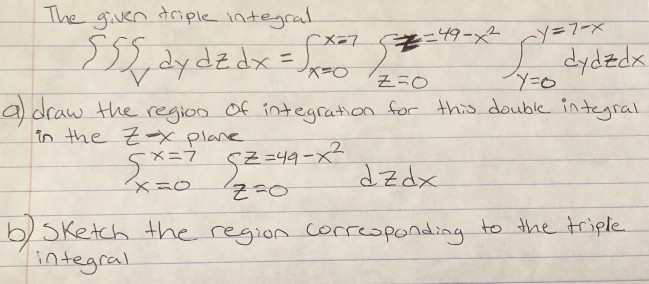x=7 dy dz dx = x Z=0 The given triple integral sss =49-x² Y = 7-8 L dydzdx a) draw the region of integration for this double integral in the Zx plane 5*=7 62-4 dzdx z=0 b) Sketch the region corresponding to the triple integral SZ=49-x²

• ### (a) Evaluate the double integral 4. (sin cos y) dy dr. Hint: You may need the formula for integration by parts (b) Show...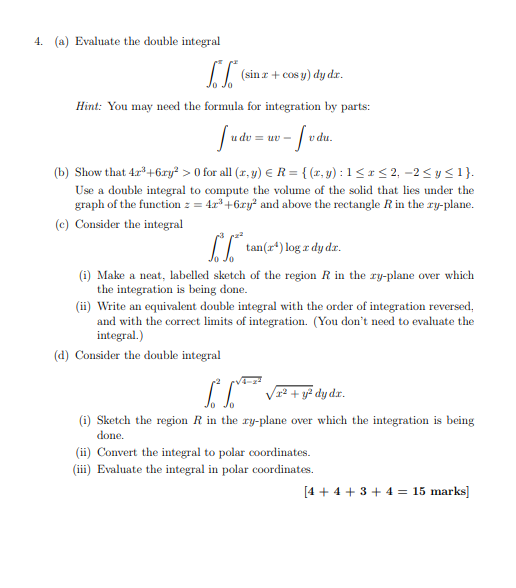(a) Evaluate the double integral 4. (sin cos y) dy dr. Hint: You may need the formula for integration by parts (b) Show that 4r+6ry>0 for all (r,y) ER-(x,y): 1S2,-2Sysi) Use a double integral to compute the volume of the solid that lies under the graph of the function 4+6ry and above the rectangle R in the ry-plane. e) Consider the integral tan(r) log a dyd. (i) Make a neat, labelled sketch of the region R in the ry-plane over...